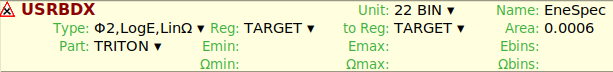# Plot error with USRBDX

Dear FLUKA expert,
The attachment is my file ,I want to get Triton’s energy spectrum with USRBDX.
At plot interface,I give all parameters default and plot ,it returns error “line 0：all points y value undefined”.could you help me to find where is the problem?
LiF.flair (2.9 KB)

Dear @xiongbp,

The error is telling you that you are not scoring anything.
Why? IIn the USRBDX card you can see that you
used the same region for your WHAT5 (‘reg’ in Flair) and WHAT6 (‘to reg’):As you can observe in this presentation (pag 4), USRBDX scores average double-differential fluence or current of a given type or family of particles on a given surface. Then, you have to define from which region (WHAT5) to which region (WHAT6) you want to do the scoring. If WHAT5=WHAT6 the fluence or current will be zero.

Thus, Changing ‘to reg’ → ‘VOID’ should solve your problem.

Let me know if you need more help.
Best,

André

3 Likes

Dear André,
Thank you,it works,but I have other question here is the angular.my geometry is cylinder,if I want to score the energy spectrum at different surface separetely.How should I set omega and type?
Actually,I am not understand omega and phi clearly.if omega is the angular between particles’ direction and the surface,the omega should be -pi/2 to pi/2,but omega(min) must belong to (0,2pi)?

Dear @xiongbp,

The WHAT1 Allows you to select the following options for your scoring:

Phi: Here you score fluence. 1 or 2 depends on if you want to score one-way (In your case, particle moving from ‘TARGET’ to ‘VOID’) or two-ways (you score also particle coming from ‘VOID’ to ‘TARGET’)

I: Here you score current. 1 or 2 is the same as I explained above.

E: Energy, as you know

Omega: the solid angle, i.e. the integral of ( sin(theta) x dtheta x dphi ). What you defined as “the angle between particles direction” is theta. A proper definition of theta would be:

“The angle between the particle direction and the normal to the surface”,

The range of theta depends on the selection of one-way ( [0, pi/2] rad ) or two-ways ( [0, pi] rad ) , getting an Omega in [0, 2pi] or [0,4pi] sr (steradian).

A comment also related to this, see the manual, which explains that the angular distribution must be intended as distributions in solid angle 2pi (1-cos(theta)), and you can get more info related to this scoring card.

In addition, observe that you can define the range of Omega depending on your interest, choosing Omega_min and Omenga_max, and the correspondent number of bins

Finally, there is no way to score what you need (different surfaces) only with one USRBDX card. I recommend you to create new regions, in order to define properly the surfaces where you want to obtain the fluence and define a new USRBDX card for each surface.

Does it make sense to you?

Cheers,
André

3 Likes

2 posts were split to a new topic: Understanding USR-1D plot